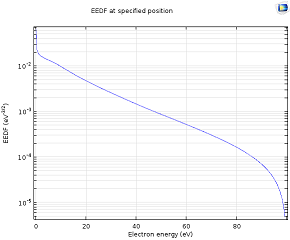# Application Gallery

## Boltzmann DC Glow Discharge

Application ID: 35491

This application models a DC glow discharge. The electron energy distribution function (EEDF) and electron transport properties are computed with the Boltzmann Equation, Two-Term Approximation interface. Since input parameters for the Boltzmann Equation, Two-Term Approximation interface, like the ionization degree of the plasma, are not known a priori, an iterative process is performed. A Boltzmann study and a plasma study are computed alternately until the deviation in electron density falls below a user-defined value. As a result, the EEDF at each point of the geometry can be examined.This model example illustrates applications of this type that would nominally be built using the following products: